×

Html & Html5

Introduction to Html

Component of Html

Html Form

Html5 basics

Html5 Grid

Html5 Graphics

Html5 Media

Html5 APIs

Python and Pandas

Introduction to Python

Component of Python

Python Sets

Sets :- A set is a collection of data type that is iterable ,mutable(changeable) ,and has no duplicate elements,and collection is unordered and unindexed. In Python sets are written by curly bracket {}.
Examples:-

Create a set

thisset = {'Banana', 'Mango', 'Apple'}

print(thisset)

Output of this Program

So here in above program we had given the command of creating Set data to our Python interpreter. Let’s see .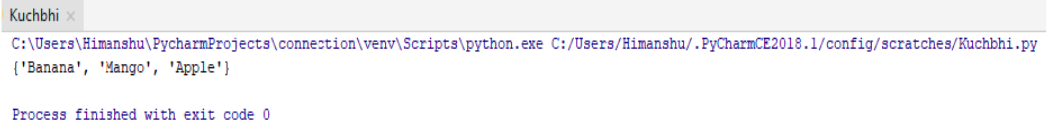So our ‘Set’ is been ready.

Access Items in Sets

Here in the Sets data type ,we cannot access item in sets by referring to an index, because sets is unordered the item has no index .

Let’s understand this by an example :-

thisset ={'Banana', 'Mango', 'Apple'}

print(thisset)

Output of this Program

So here in the above program we just want to access only one item from the sets who is having index no. 1, means ‘Apple’ we want because first one is included ‘0’ in Python.
So let’s see what output it gives :-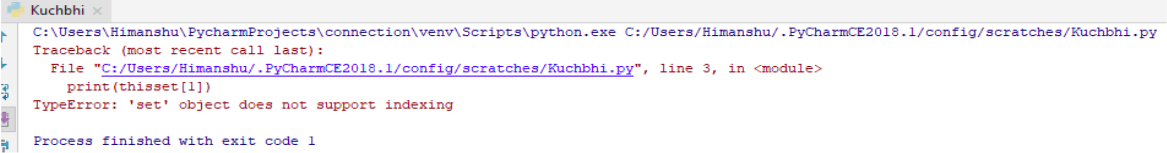It gives the error because it does not support indexing

Change Set values

Changing the items in set is not possible . If a set is been created than you can’t perform any changes into the Sets.

Let’s understand this by an example :-

thisset ={'Banana', 'Mango', 'Apple'}

thisset='Guava'

print(thisset)

Output of this Program

So here in the above Program we just gave command to our Python interpreter that we want to change the item , of index number ‘2’ from ‘Mango’ to ‘Guava’ .
So let’s see what output it gives :-It gives the error because once the Set is created than it can’t change but addition we can do here .

Loops through Sets

In Sets we can use ‘for’ loop through the specified values present in sets by using the ‘in’ keyword.

Let’s understand this by an example :-

thisset={'Mango' , 'Apple' ,'Banana'}

for X in thisset:

print(X)

Output of this Program

So here in the above program we just gave command to our Python interpreter that we want to print all the items , one by one .
So let’s see what output it gives :-Here it just shows all the elements one by one .

( More about Loops we will talk further on the looping section. )

Check if item or element exist in Sets

We can also check items or elements in our sets by using the ‘in’ keyword.
Let us understand this by the following example :-

thisset = {'Banana', 'Mango', 'Apple'}

if 'Mango' in thisset :

print("Yes it is in thisset")

Output of this ProgramSo our ‘Mango’ is in the set . So it just give the correct output.

( We will read in detail regarding ‘if’ statement . )

Methods in Sets

As we know that methods are basically the function which we want to perform in our coding . In Python there are some inbuilt methods are there for Sets too .
So let’s see some of them :-

The len() method - It just returns the length of the Sets

thisset = (‘Banana’, 'Apple', 'Mango')

print(len(thisset))

Output of this Program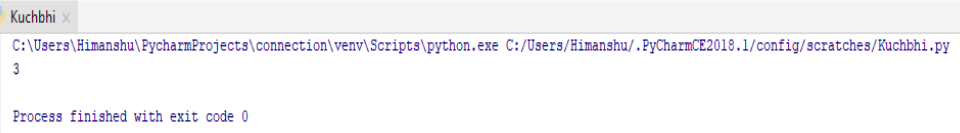So here we just get the length of the set based on their index number.

• When you add one item of Set use to add() method.

• When you add more than one item to Set use to update() method.

thisset = {'Banana', 'Mango', 'Apple'}

print(thisset))

Output of this Program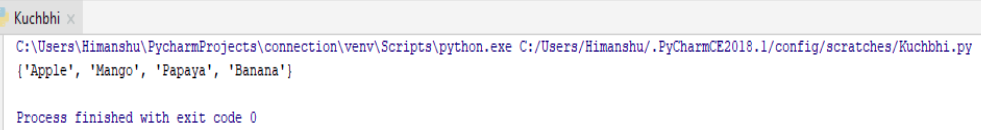Add multiple Items to a Set, Using the update() method:-

thisset = {'Banana', 'Mango', 'Apple'}

thisset.update(['Papaya','Orange', 'Cherry'])

print(thisset))

Output of this ProgramSo here through update() method we add many elements to our set.

Removing items on sets

For removing the item on Sets, we can use to remove() or discard ()method.

To remove the item of set,using the remove() method .

thisset={'Banana','Mango' , 'Apple'}

thisset.remove('Mango')

print(thisset)

Output of this Program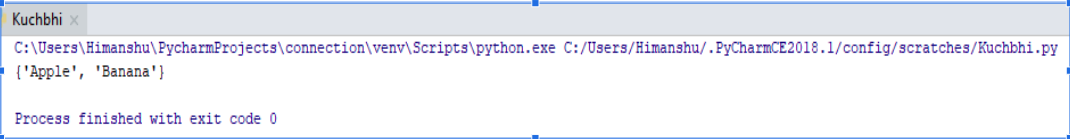So here it just remove the ‘Mango’ . But if the item remove does not exist ,remove() will rise an the error.

To remove the item of set,using the discard() method .

thisset={'Banana','Mango' , 'Apple'}

print(thisset)

Output of this ProgramSo here it just remove the ‘Mango’ . But here if the item discard does not exist ,discard() will not rise any error.

The set constructor , set() can used to make a set

thisset = set(('Banana', 'Mango', 'Apple')) # note the double round brackets

print(thisset)

Output of this Program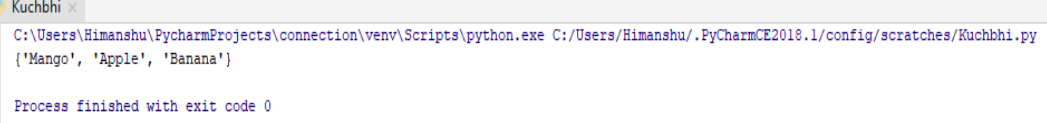So here through set() constructor our set is ready.

Some methods of Sets and their functionality

Python has a Set of built-in methods that can use on Sets.

Method Description
clear() Remove all the element to the set
copy() Return a copy of the set
difference() Return set containing between two and more sets.
difference update()) Remove the item of Sets and they include other specified
intersection() Return a set, and intersection two other sets.
intersection update() Remove the item of this sets that are not present in other specified set()
isdisjoint() Return whether two set intersect or not.
issubset() Check if the Set is Subset of another Set.
issuperset() Check if the Set is Superset of another set
pop() Removes an element from the Set.
set() Return of sets.
remove() Removed the specified element.
symmetric difference() Return Symmetric difference
symmetric difference update() Update Set with Symmetric difference
union() Return a set containing of union set
any() Check if any Element iterable true.
all() Return true when all element iterable is true.
ascii() Return String Containing Printable Representation.
bool() Convert values of boolean.
enumerate() Return the enumerate object.
filter() Construct iterator from element which are true.
frozenset() Return immutable frozenset for object.
iter() Return iterator for an object.
max() Returns largest element.
min() Returns smallest element.
len() Returns length of element.
map() Applies function and returns a list.
sorted() Returns the sorted list given iterable.